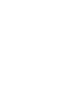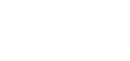## Questions

1.
1.
1. State coulombs law of electrostatics force.
2. Define capacitance.
2. Describe how the type of charge on a charged metal rod can be determined.
3. The figure below shows a hollow negatively charged sphere with metal disk attached to an insulator placed inside. State what would happen to the leaf of an uncharged electroscope if the metal disk were brought near the cap of electroscope. Give a reason for your answer.4. State two ways of charging the magnitude of the deflection of the leaf of an electroscope.
5. The figure below shows an arrangement of capacitors connected to a 10v. D.C supply determine:-1. The charge stored in the 2μF capacitor
2. The total capacitance of the arrangement
2. The figure below represents two parallel plates of a capacitor separated by a distance d. Each plate has an area of A square units. Suggest two adjustments that can be made so as to reduce the effective capacitance.3. The figure below Shows part of a circuit containing three capacitors. Write an expression for CT. The effective capacitance between A and B.4. State the law of electrostatic charge.
5.
1. The capacitors in the circuit in the figure below are identical and initially uncharged.Switch S1 is opened and switch S2 closed. Determine the final reading of the voltmeter, V.
2.In the circuit diagram shown in the figure above each cell has an e.m..f of 1.5 and internal resistance of 0.5Ω. The capacitance of each capacitor is 1.4μF.
When the switch s is closed determine the:
2. Charge on each capacitor
6. A 2μF capacitor is charged to a potential of 200V, the supply is disconnected. The capacitor is then connected to another uncharged capacitor. The p.d. across the parallel arrangement is 80V. Find the capacitance of the second capacitor.
7. A 5μF capacitor is charged to a p.d of 200v and isolated. It is then connected to another uncharged capacitor of 10μF. Calculate
1. The resultant p.d
2. The charge in each capacitor.
8. Three capacitors of 1.5μF, 2.0 μF and 3.0 μF are connected in series to p.d. of 12V. Find;-
1. The combined capacitance.
2. The total charge stored in the arrangement
3. The charge in each capacitor.
9. In the circuit of the figure below C1=2 μF, C2 =C3 = 0.5 μF and E is a 6V battery. Calculate the total charge and p.d across C110. In an experiment to study the variation of charge stored on capacitor and the potential difference across it, the following results were obtained.
 Charge, Q (μC) 0.08 0.16 0.24 0.32 0.4 0.56 p.d (v) 2 4 6 8 10 14

1. Plot a graph of charge Q. against p.d
2. Use your graph to determine:-
1. Capacitance of the capacitor.
2. Energy stored in the capacitor when the p.d across its plate is 10V.

1.
1.
1.
2. Ability to store charge given by the quality of charge it can store per unit p.d
2. Bring it near a charged electroscope (say +ve). If there is divergence then the rod is +vely charged. If not, charge the electroscope -vely and bring the rod near. If divergence is observed then they have the same charge.  Note that if decrease in divergence is observed in both cases then the rod is simply a conductor and its not charged.
3. Nothing would happen to the leaf of the electroscope. This is because in a hollow charged conductor, the charges are distributed on the surface of the charged conductor and not inside.
4. Earthing or using another charged body
1. Q= CV
= 2 x 10
= 20 μC
2. Series = 1/C = 1/3 + 1/3
1/C = 2/3
C= 3/2 = 1.5 μ F
Parallel Ct = 1.5 + 2
= 3.5 μF
2. - By moving one plate sideways so as to reduce the area of overlap.
- By increasing the separation distance, d
3. Parallel = CT = C1 + C2
Series = 1/CT = 1/C3 + 1/(C1+ C2)
1/CT = (C1 + C2) + C3
C3(C1 + C2)
CTC3 (C1 + C2)
C1 + C2 + C3
4. Like charges repel while unlike charges attract.
1. When S1 is closed V= 3V
Charge stored in C2 is Q = CV = 3C
When S1 is opened, ½Q is transferred from C1 to C2
Since they are in parallel p.d = 1.5V
2.
1. RT = 6 + 5 + 0.5 = 11.5 Ω
∴ I =  E = 3.0 = 0.26A
RT   11.5
2. Q= CV = 1.4 x 3
= 4.2 μC
5. QT = CV = 400 μC
CT = (2 + x)
QT = 80
(400μC) = 80
(2+x)
x= 3 μF
6.
1. Ct = (5+ 10) μF = 15 μF
Q= 200 x 5
= 1000 μC
Q/V= 15 μF
1000 = V
15
V= 66.7V
2. Q/V = C
Q/66.7= 5
Q= 66.7 x 5
= 333.5 μF
Q/V = 10
Q/66.7   = 10
Q= 66.7 x 10
= 667 μF
7.1. 1/CT = 1/1.5 + ½ + 1/3
= 0.67 + 0.5 + 0.33
1/CT = 1.5
CT= 0.67 μF
2. Q= CTV
= 0.67 x 12
Q= 8.04 μC
3. 8.04 μC this is because they are in series arrangement therefore the quantity of charge is equal in all the capacitors.
8. For parallel CT = C2 + C3
= 1.0 μF
Series CT = product/sum = 2/3 = 0.667 μF
QT = CV = 2/3 x 6 = 4 μC
QC1 = CV = QT = 4 μC
V= QT/C1 = 4/2 = 2V.
9.
1. Graph of change against p.d
2. Q= CV
C= gradient = 0.24 – 0.08
6.0 – 2.0
= 0.16
= 4.0
= 0.04 μF
3. Energy stored = ½ QV = area
= ½ x 40 x 10-6
= 2.00 x 10-4J.

• ✔ To read offline at any time.
• ✔ To Print at your convenience
• ✔ Share Easily with Friends / Students